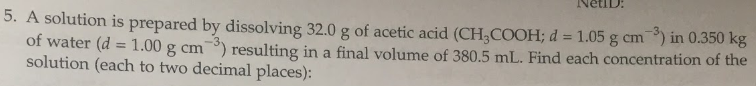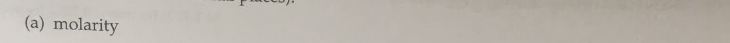# 5. A solution is prepared by dissolving 32.0 g of acetic acid (CH3COOH; d = 1.05 g cm^-3) in 0.350 kg of water (d = 1.00 g cm^3) resulting in a final volume of 380.5 mL. Find each concentration of the solution (each to two decimal places): (a) molarity